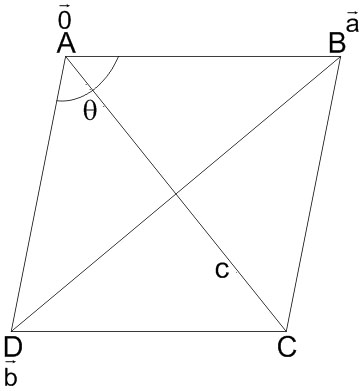#### In    a    parallelogram,Option 1)Option 2)Option 3)Option 4)As we learnt in

Scalar Product of two vectors (dot product) -- whereinis the angle between the vectorsThus let A be positionvector of B is, D is..............(i)

Also here,Put in (i)Option 1)This option is correct.

Option 2)This option is incorrect.

Option 3)This option is incorrect.

Option 4)This option is incorrect.

#### Plabita